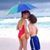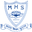Trending ▼   ResFinder# ISC Class XI Prelims 2018 : Mathematics (Modern High School for Girls (MHS), Kolkata)

6 pages, 17 questions, 0 questions with responses, 0 total responses,00Norman Thendup Modern High School for Girls (MHS), Kolkata
+Fave Message
 Home > ronajman >   F Also featured on: School PageFormatting page ...

MATHEMATICS (Three hours) (Candidates are allowed additional 15 minutes for only reading the paper. They must NOT start writing during this time.) --------------------------------------------------------------------------------------------------------------------The Question Paper consists of three sections A, B and C. Candidates are required to attempt all questions from Section A and all questions EITHER from Section B OR Section C Section A: Internal choice has been provided in three questions of four marks each and two questions of six marks each. Section B: Internal choice has been provided in two questions of four marks each. Section C: Internal choice has been provided in two questions of four marks each. All working, including rough work, should be done on the same sheet as, and adjacent to the rest of the answer. The intended marks for questions or parts of questions are given in brackets [ ]. Mathematical tables and graph papers are provided. --------------------------------------------------------------------------------------------------------------------- SECTION A (80 Marks) Question 1 [10x2] (i) Let : be a function defined by ( ) = is one-one but not onto. (ii) Find the domain and range of the function ( ) = [ ]. (iii) Find the square root of complex number 11 60 . (iv) For what value of will the equations 2 21 = 0 2 3 + 35 = 0 have one common root. (v) In a , show that ( + ) cos = 2 where = (vi) Find the number of ways in which 6 men and 5 women can dine at a round table if no two women are to sit together. (vii) Prove that sin 20 sin 40 sin 80 = , where . Then show that + + 2 3 8 (viii) If two dice are thrown simultaneously, find the probability of getting a sum of 7 or 11. | 2| (ix) Show that lim (x) Find the point on the curve 2 = 4 , the tangent at which is parallel to the straight line = 2 +4. 2 2 does not exist. SPECIMEN PAPER ISC 2018 CLASS XIFormatting page ...Formatting page ...Formatting page ...Formatting page ...Formatting page ...